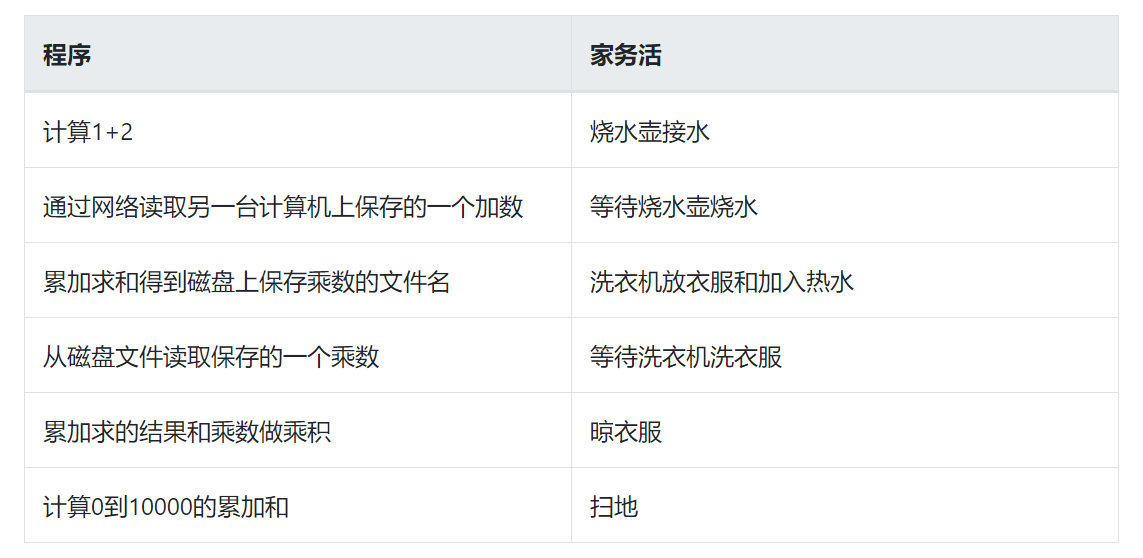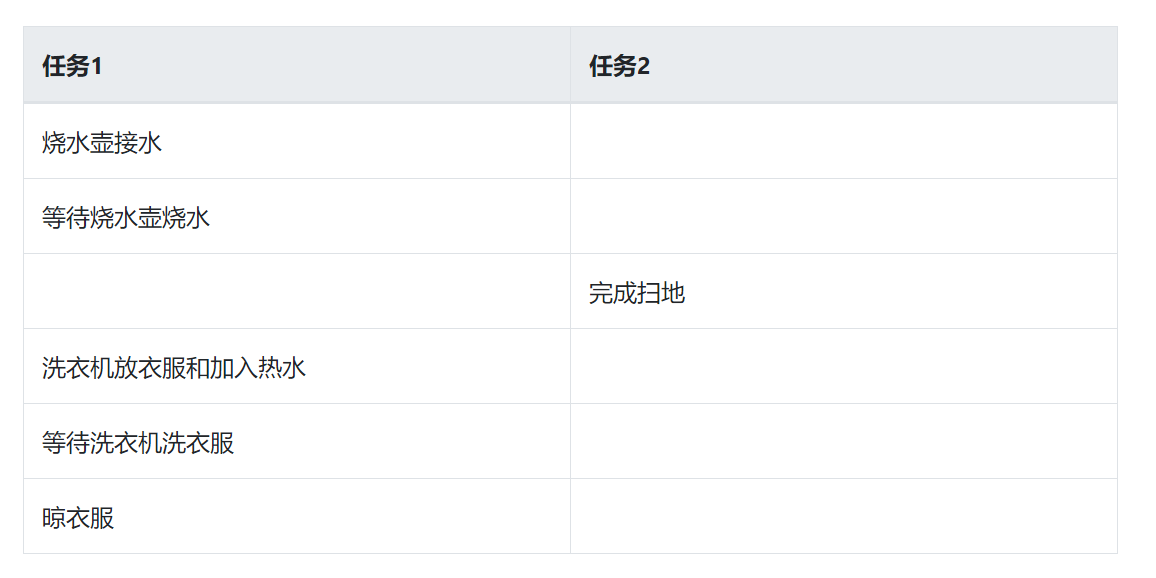# 家务活中的 python 协程• 烧水壶接水

• 等待烧水壶烧水

• 洗衣机放衣服和加入热水

• 等待洗衣机洗衣服

• 晾衣服

• 扫地

### 2. 程序描述def get_network_number() -> int:  """通过网络获取一个整数"""   ... def get_file_number(filename: str) -> int:   """读取磁盘文件获取一个整数"""   ... def cumulative_sum(start: int, end: int) -> int:   """累加求和"""   sum = 0   for number in range(start, end):     sum += number     return sum def task():   """任务"""   result = 1 + 2   network_number = get_network_number()   result += network_number   file_number = get_file_number(f"{result}.txt")   result *= file_number   sum = cumulative_sum(0, 10000) task() 

### 3. 问题分析及程序改进

from threading import Thread def get_network_number() -> int:   """通过网络获取一个整数"""   ... def get_file_number(filename: str) -> int:   """读取磁盘文件获取一个整数"""   ... def cumulative_sum(start: int, end: int) -> int:   """累加求和"""   sum = 0   for number in range(start, end):     sum += number     return sum def task1():   ""任务1"""   result = 1 + 2   network_number = get_network_number()   result += network_number   file_number = get_file_number()   result *= file_number def task2():   """任务2"""   sum = cumulative_sum(0, 10000) t1 = Thread(target=task1) t2 = Thread(target=task2) t1.start() t2.start() t1.join() t2.join() 

### 4. 引入协程import asyncio # io任务改为协程 async def get_network_number() -> int:  """通过网络获取一个整数"""   ... # io任务改为协程 async def get_file_number(filename) -> int:  """读取磁盘文件获取一个整数"""   ... # 计算密集型任务不用改协程 def cumulative_sum(start: int, end: int) -> int:   """累加求和"""   sum = 0   for number in range(start, end):     sum += number     return sum async def task1():   """任务1"""   result = 1 + 2   network_number = await get_network_number()   result += network_number   file_number = await get_file_number(f'{result}.txt')   result *= file_number async def task2():   """任务2"""   sum = cumulative_sum(0, 10000) async def main():   task1 = asyncio.create_task(task1())   task2 = asyncio.create_task(task2())   await task1   await task2   asyncio.run(main()) 

async def cumulative_sum(start: int, end: int):   result = 0   for i in range(start, end):     if i % 100 == 0:     await asyncio.sleep(1)     result += i     return result async def task2():   """任务2"""   sum = await cumulative_sum(0, 10000) 

### 5. 任务和事件

• 任务

• 事情

async def task1():   """任务1"""   result = 1 + 2   network_number = await get_network_number()   result += network_number   file_number = await get_file_number(f"{result}.txt")   result *= file_number 

1. result = 1 + 2

2. result += network_number

3. result *= file_number

async def task2():   """任务2"""   sum = await cumulative_sum(0, 10000) 

async def cumulative_sum(start: int, end: int):   result = 0   for i in range(start, end):     if i % 100 == 0:       await asyncio.sleep(1)   result += i   return result 

### 7. 细节讨论

async def get_file_number(filename):   with open(filename) as f:     number = int(f.read())     return number 

import asyncio def _read_file(fd):   return fd.read() async def get_file_number(filename):   loop = asyncio.get_event_loop()   with open(filename) as f:   number = await loop.run_in_executor(None, _read_file, f)   return int(number) 

### 8. 协程的用武之地

1）为什么磁盘 IO 需要用线程调度而网络 IO 不需要？

2）引入线程改造后的协程不是还会存在频繁的任务切换浪费 CPU 时间吗，这样做效率会比多线程方式高吗？

• 网络编程中有同步和异步的方式，异步的方式就是 IO 多路复用。

• IO 多路复用支持的文件描述符类型和操作系统有关。

• python 协程中任务的切换依赖于 IO 多路复用。

• Windows 下磁盘 IO 不支持 IO 多路复用，即便有操作系统支持，如果标准库未做封装，需要我们自己封装。

• 如果程序中未涉及网络 IO，那么使用协程并不能有效的降低任务切换的开销，但协程良好的同步编程方式依旧可用。

• 不同的编程语言对协程的实现有所不同，使用方法和应用场景也不尽相同。## 评论